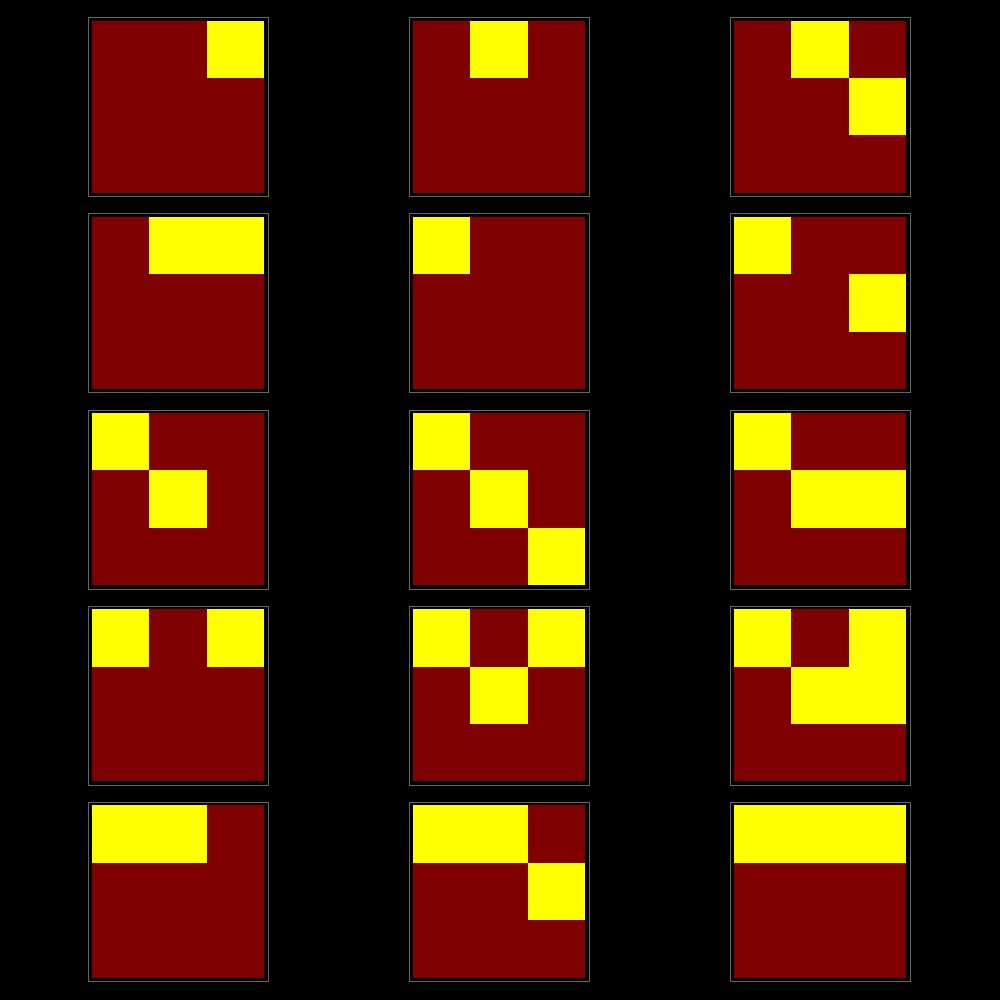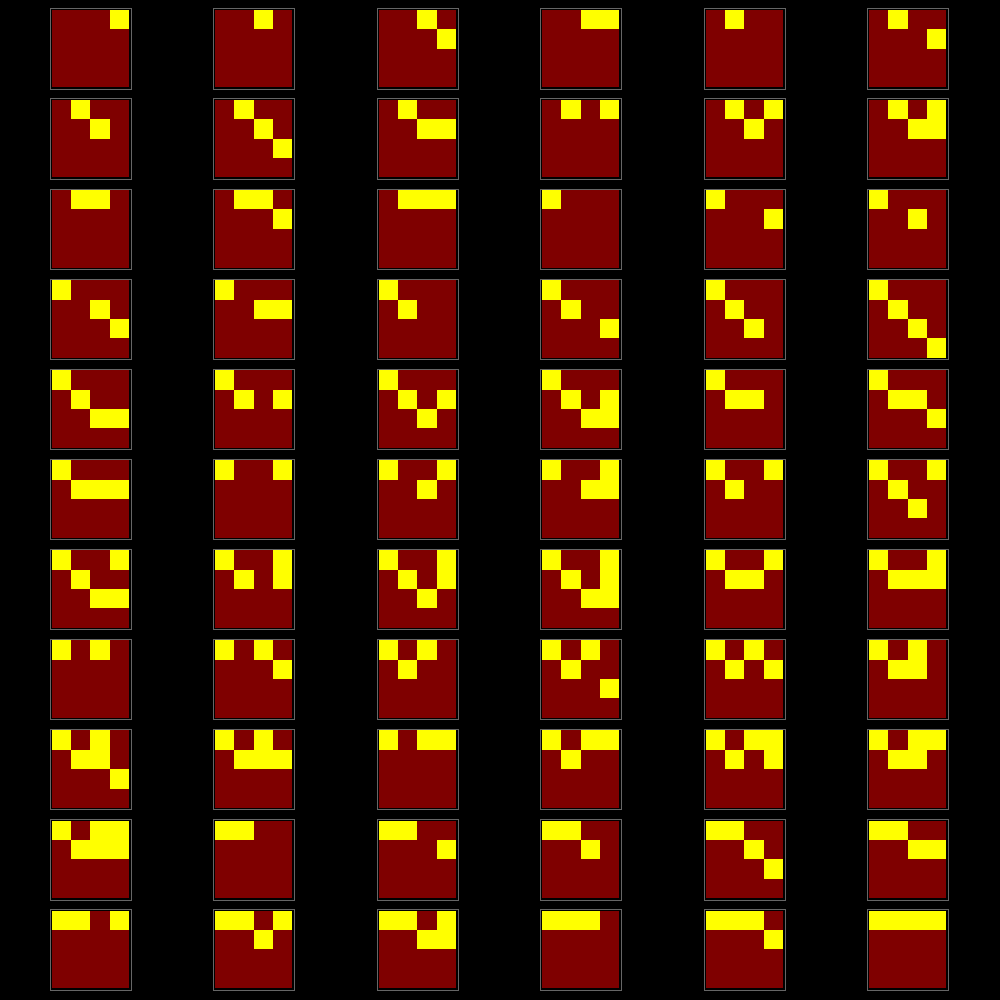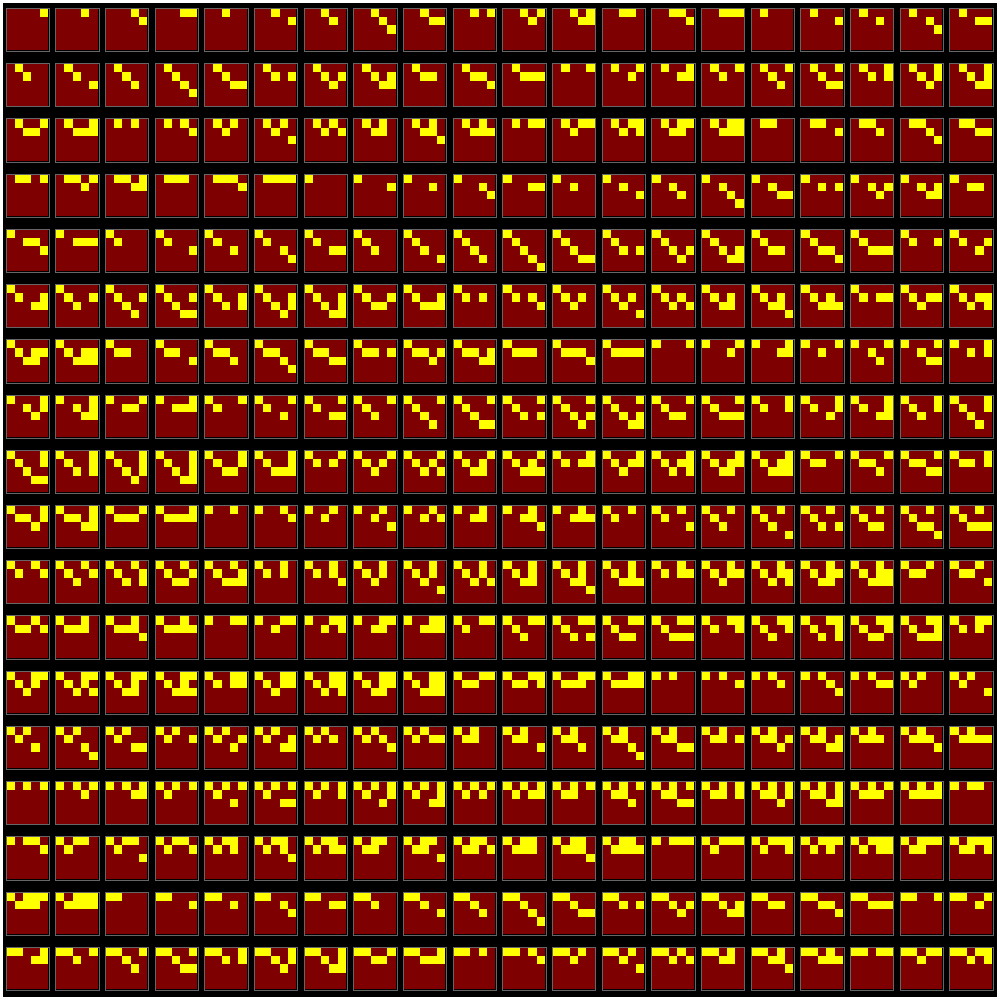M A T H 2 1 B
Mathematics Math21b Spring 2016
Linear Algebra and Differential Equations
Exhibit: How many row reduced echelon matrices?
 The number of row reduced n x n matrices is given by the sequence A006116, a sum of Gaussian Binomial coefficients. It is the number of subspaces of a vector space over the field Z2 or the number of distinct binary linear codes of length n or the number of Abelian groups in C2n. The sequence starts as follows: ```1, 2, 5, 16, 67, 374, 2825, 29212, 417199, 8283458, ... ``` Here are all 15 nonzero 3x3 cases:Here are all 66 nonzero 4x4 cases. Would be nice homework problem....And counting all the 5x5 cases would be a nice exam problem...Here is how one can compute that with brute force: ```(* How many row reduced 0-1 matrices are there of size n x n ? *) F[n_]:=Module[{i,u,m=n^2}, i[x_]:=Partition[PadLeft[IntegerDigits[x,2],m],n]; u=Map[i,Range[0,2^m-1]]; (* produces list of all 0-1 matrices *) v=Union[Table[RowReduce[u[[k]]],{k,2^m-1}]]; w={}; Do[If[Sort[Union[Flatten[v[[k]]]]]=={0,1}, w=Append[w,v[[k]]]],{k,Length[v]}]; 1+Length[w]]; F ``` When first computing this, I (Oliver) had not checked that the row reduced matrices are still 0-1 matrices. Actually, they are not in general. Thanks to Jun Hou Fou (whom you know from teaching 21a last semester) for pointing this out to me and telling about the connection to the subspace problem and the sequence A006116. Please send questions and comments to knill@math.harvard.edu Math21b Harvard College Class Number:16325 Course ID:110989| Oliver Knill | Spring 2016 | Department of Mathematics | Faculty of Art and Sciences | Harvard University, [Canvas, for admin], Twitter